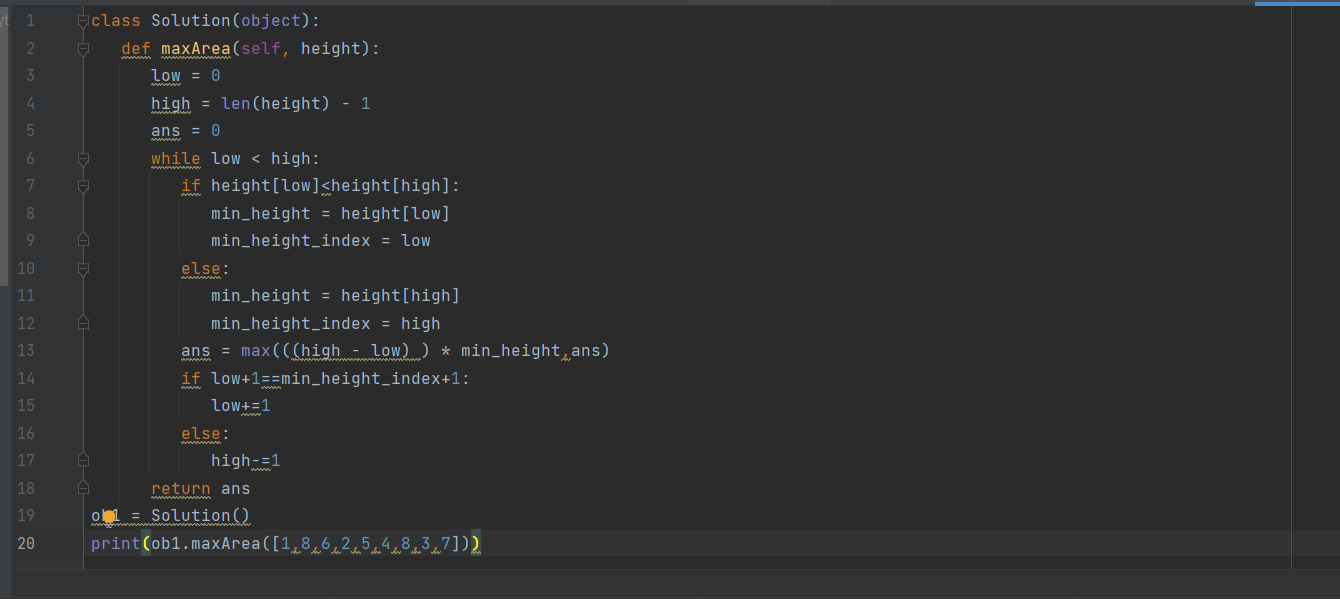• by Team Handson
• February 16, 2023

## Important FAANG Question: â€œContainer with Most Waterâ€ with Explanation and Code

An important question asked in FAANG/ MAANG interview that is You are given an integer array height of length n. There are n vertical lines drawn such that the two endpoints of the ith line are (i, 0) and (i, height[i]). Find two lines that together with the x-axis form a container, such that the container contains the most water.

Notice: You may not slant the container.

Explanation

Give n non-negative integer A1, A2, ..., An, each value represents a point at coordinate (i, a[i]). Draw n vertical lines in the coordinates, and the two endpoints of the vertical line I are (i, a[i]) and (i, a). Find the two lines, so that the containers that they and the x-axis can accommodate the greatest amount of water.Input: [1,8,6,2,5,4,8,3,7]

Output: 49

The vertical line in the figure represents the input matrix [1,8,6,2,5,4,8,8,3,7]. In this case, the maximum value of the container that can accommodate the water (indicated as the blue part) is 49.

Example 1:

Input: height = [1,1]

Output: 1

Example 2:

Input: height = [4,3,2,1,4]

Output: 16

Example 3:

Input: height = [1,2,1]

Output: 2

Solution (Python):